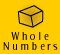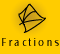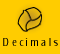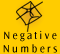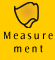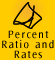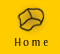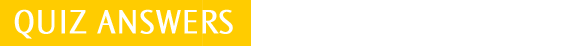| Meaning and Models | Key Ideas|
| Percent Examples | Ratio Examples | Rates Examples |

 1. a) 95/100 or 19/20; 0.95 b) 13.5/100 or 27/200; 0.135 c) 42/100 or 21/50; 0.42 d) 1/100; 0.01 e) 1/1000; 0.001 2. a) 37% b) 164% c) 50% d) 70% e) 64% 3. a) 1 % b) 83% c) 0.5% d) 110% e) 20% 4. a) ratio of boys to girls is 3 : 5 b) ratio of people who watch TV to people who don't is 9 : 1, or we could also say, ratio of people who watch TV to total number of people is 9 : 10 c) ratio of left to right handedness in class is 3 : 22, or we could also say, ratio of left handedness to the rest of the class is 3 : 25 d) ratio of rice to water is 1:2 e) ratio of the mass of rice to flour is 1 : 1 5. a) 10 : 20 = 1 : 2 = 2 : 4 b) 30 : 50 = 3 : 5 = 60 : 100 c) 100 : 100 = 1 : 1 = 2 : 2 d) 6 : 4 : 2 = 3 : 2 :1 = 30 : 20 : 10 e) 75 : 25 = 3 : 1 = 6 : 2 6. a) 3 : 3 : 4 = 3/10 : 3/10 : 4/10 = 0.3 : 0.3 : 0.4 = 30% : 30% : 40% b) 11 : 4 : 5 = 11/20 : 4/20 : 5/20 = 0.55 : 0.2 : 0.25 = 55% : 20% : 25% c) 4 : 1 = 4/5 : 1/5 = 0.8 : 0.2 = 80% : 20% d) 5 : 3 : 1 : 6 = 5/15 : 3/15 : 1/15 : 6/15 = 0.33 : 0.20 : 0.07 : 0.40 = 33% : 20% : 7% : 40% (note 0.07 has been rounded from 0.0666 recurring) 7. a) 100 metres / 15 seconds = 6.67 m/s b) 850 kilometres / 10 hours = 85 km/h c) 160 words / 2 minutes = 80 words per minute d) 600 packets / 5 minutes = 120 packets per minute

Extra questions:

1. 20% is 20 parts out of 100, so 20 rectangle out of 100 must be shaded

2.

(a) Percentage shaded = 9 rectangles out of 50, which equals 18 rectangles out of 100 which equals 18%

(b) Percentage not shaded = Total percent less the percent shaded = 100 - 18 = 82%

3. The ratio of pink to white = 2 : 6 This is the same as 1 : 3 For every one pink there are 3 white.

4. The order is important in ratio. Also, while the units must be the same, there are no units in the answer.

(a) ratio of biro length : ruler length = 23 : 30

(b) ratio of ruler length : biro length = 30 : 23

5.

 Percentage Common Fraction Decimal Fraction 32%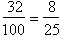Thirty two hundredths = 0.32 Six hundredths = 6%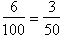0.06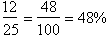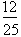Forty eight hundredths =0.48

6. (a) Length of dog = 90 cm

The dog is 3 times the length of the bird, so bird is 30 cm.

(b) The ratio of dog : bird = 90 : 30. This is the same as 3 : 1. So, for every 1 cm of the bird there are 3 cm of the dog.

7.
(a)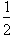kilogram = 500 g. Hence, ratio of sugar to butter = 300 : 500. This is the same as 3 : 5 For every 3 units of
sugar there are 5 of butter.

(b) Ratio of butter to sugar = 500 : 300 this is the same as 5 : 3

Distance is measured in km and time is measured in hours. Hence the two quantities being measured are distance and time.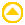1. a) 33 1/3% = 1/3 = 0.33 b) 231% = 231/100 = 2.31 c) 0.03% = 3/10000 = 0.0003 d) 75% = 75/100 = 3/4 = 0.75 e) 12.5% = 12.5/100 = 0.125 2. Two methods have been used to calculate these answers. Either method can be used for each question. The 20% discounted prices are: a) \$2.30 x 0.8 = \$1.84 b) \$1 900 x 0.8 = \$1 520 c) \$6.50 - (0.2 x \$6.50) = \$5.20 d) \$11 - (0.2 x \$11) = \$8.80 e) \$40 000 x 0.8 = \$32000 f) \$30 x 0.8 = \$24 3. Two methods have been used to calculate these answers. Either method can be used for each question. The prices including the 15% increase are: a) \$2.30 x 1.15 = \$2.65 b) \$1 900 x 1.15 = \$2 185 c) \$6.50 + (0.15 x \$6.50) = \$7.48 d) \$11 + (0.15 x \$11) = \$12.65 e) \$40 000 x 1.15 = \$46000 f) \$30 x 1.15 = \$34.50 4. a) 9% of 70 is nearly 10% so that is about 7 b) 48.5% of 120 is nearly 50% so that is about 60 c) 24% of 200 is nearly 25% (which is one quarter) so that is about 50 d) To find 0.5% of 100, I know 1% of 100 is 1, so 0.5% would be 0.5 exactly e) 98% of 163 is nearly 100% so that is about 160 (or we could about say 163) f) 19% of 5000 is nearly 20% (which is one fifth) so that is about 1000 g) 47% of 820 is nearly 50% so that is about a half of 820 which is 410 h) 69% of 8960 is a bit over two thirds or nearly 70% of 8960. If we round up 8960 to 9000 and then find two thirds of it, that is about 6000 5. a) 6 : 12 = 1 : 2 = 18 : 36 b) 1 : 3 = 2 : 6 = 5 : 15 c) 2 : 7 = 4 : 14 = 8 : 56 = 1 : 3.5 d) 7 : 22 : 25 = 14 : 44 : 70 e) 3 : 9 : 24 : 36 = 1 : 3 : 8 : 12 f) 5 : 10 : 26 = 10 : 20 :52 a) 14 : 6 : 8 = 7 : 3 : 14 b) 2 : 50 000 = 1 : 25 000 c) 4 : 12 : 48 = 1 : 3 : 12 d) 30 000 : 3 = 10 000 : 1

Extra questions:

1. Discount = 12% of 864 =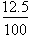x 864 = 0.125 x 864 = 108

Amount paid for TV = Marked Price - Discount = 864 - 108 = 756

The discounted price of the TV = \$756

2.
(a) The units must be the same. 1 day = 24 hours

12 hours is to 1 day = 12 : 24 = 1 : 2 ( because 12 divides evenly into 12 and 24)

(b) The units are the same. Multiply both fractions by 7 to remove the denominator of the fractions. The answer is 3 : 5.
3. The given ratio, 3 : 4, can be written as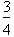. Since this fraction is less than 1, when we multiply it by 24 g the number of grams will be less than 24.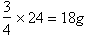Therefore the amount of fat in light and healthy Brand A ice-cream is 18g.

4. Total number of units = 7 + 3 = 10

Jane receives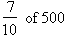=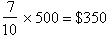5. The scale is 1 : 100 000, so multiply by 10,

10 x 1 : 10 x 100 000 = 10 : 1 000 000

The towns are 1 000 000 cm apart

This means the towns are 10 000 m apart. (There are 100 cm in a metre)

This means the towns are 10 km apart. (There are 1000 m in a kilometre)

6. 20 beats in 5 seconds.

Divide both the 20 and the 5 by 5

This gives 4 beats in 1 second i.e. 4 beats/s

7. The front width of the drawing of the house is 6 cm. The width of the front of the real house is 6 x 200 = 1 200 cm = 12 m.

In one year’s time the value of the investment =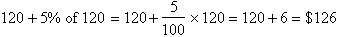At the end of two years, the value of the investment =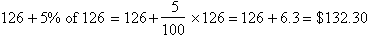1. a) 80% of 45 seats is 36 seats b) 19 out of 45 seats is 42.2% 2. 13 girls is 45% of the class. 13/b = 45/100 b = 1300/45 = 28.89 There are 29 students in the class 3. a) If Georgie answered 35 out of 40 questions correctly she answered 87.5 % correctly. 35/40 = r /100 r = 3500/40 = 87.5 % b) As Georgie scored above 85% she did pass c) p/40 = 97/100 p = (97 x 40)/100 = 38.8 Georgies answered 39 questions correctly 4. 300 gs is 15% of the puppy's total body weight. 300 / b = 15/100 b = 30 000 / 15 b= 2000 The puppy's total body weight is 2000 grams (2 kg) 5. a) 5 tickets out of 15 tickets is one third or 33.3% b) Ryan bought the tickets for \$65 each and then increased the cost by 10%. \$65 + 0.10 x \$65 = \$71.50 Therefore he is selling the tickets for \$71.50 c) Ryan reduces the \$65 tickets by 20%. \$65 x 0.8 = \$52 d) Ryan finally sold 73% of 15 tickets 73/100 = p/15 p = (73 x 15) / 100 p = 10.95 (we round 10.95 to 11 because 10.95 tickets does not make sense) This means Ryan sold 11 tickets. 6. The full price of the jeans was \$79. They are now discounted by 25% \$79 x 0.75 = \$59.25 The jeans now cost \$59.25 The full price of the T-shirt was \$35. It is now discounted by 25%. \$35 x 0.75 = 26.25 The T-shirt now cost \$26.25 7. a) 8 workers out of 35 workers ride their bikes. This is 22.86% b) 14 workers out of 35 workers catch public transport. This is 40% c) 13 workers ride or walk to work. This is 37.14% of the 35 workers. d) 25 out of 35 workers do not use their car to get to work, This is 71.43% e) Since the percents represent fractions out of 100 we can say; bike=23 (rounded from 22.86%) public transport =40 drive=29 (71% - rounded - do not use their car, so we can say 29% do use their car) walk=8 (3 out of 35 is about 8%) 8. The 440 g can has 35 parts out of 100 that contain fat. The 1 kg can contains 20 parts out of 100 that contain fat. A bowl of each contains the same parts out of 100 of fat as the cans, so a bowl from the 440 g will contain more fat than a bowl from the 1 kg can.

Extra questions:

1.

Fraction of Australians who attended the 2000 Olympic Games =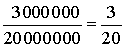andPercent of Australians who attended the 2000 Olympic Games = 15%

2.

Percent of people who prefer skiing = 100 - 28 = 72%

Fraction of people who prefer skiiing =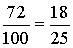3.

Fraction who liked P.E. best =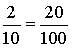Percent who liked P.E. best = 20%

4.5.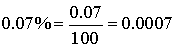6.
(a)

Commission = 15% commission of \$1 700

10% of 1 700 = 170

5 % of 1 700 = half of 10% = half of 170 = 85

15% = 10% + 5% = 170 + 85 = 255

Commission = \$255

(b) Total amount earned = 520 + 255 = \$775
7.
(a) The 15% profit on \$180 = the 15% loss on \$180, so altogether she did not make a profit or loss on the sale of the two racquets.
(b) She made \$0 on the sale of the two racquets, because they cost her \$360 and she received \$360 for them.
8.

No. of boys in Mrs Smith's class = 13

No. of boys in Mr. Jones' class = 30 - 3 = 27 (There are 10% of 30 girls = 3 girls)

Total no. of boys in the two classes = 13 + 27 = 40

Total no. of children in both classes = 20 + 30 = 50

Fraction boys =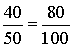Percent of boys = 80%1. a) baby : mug = 3 : 8 b) baby : regular = 3 : 20 c) mug : regular = 8 : 20 = 2 : 5 d) mug : baby = 8 : 3 2. a) turtles : fish = 2 : 22 = 1 : 11 b) ratio of orange fish to black fish is 7 : 12 c) ratio of turtles to black fish is 2 : 12 = 1 : 6 d) mottly fish : total number of fish = 3 : 22 e) mottly fish : turtles = 3 : 2 f) ratio of black fish to orange fish is 12 : 7 3. a) ratio of number of lasagnas to number of pizzas is 5 : 13 b) 13 pizzas cut into 5 pieces means that there are 13 x 5 pieces in total. Ratio of pieces of pizza to lasagnas is (13 x 5): 5. This is 13:1. You can check it by multiplying 13 x 5 to get 65 and then dividing by 5, but it is important to know this important relationship. 4. a) 1 : 1000 b) 1 : 300 c) 1 : 100 000 d) 15 : 50 000 = 3 : 10 000 5. a) smaller b) larger c) smaller d) smaller e) smaller f) larger 6. The 1.25 litre bottle is cheaper than the 600 ml bottle since it contains more than double the quantity but is less than double the price. Two of the 1.25 litre bottles would contain 2.4 litres but would only cost 15 cents more (\$3.70) than the 2 litre bottle. Therefore the 1.25 litre bottle is the best value. You could also solve this problem by working out the cost per litre for each bottle of water. The 1.25 litre bottle is the best value at \$1.48 per litre1. a) 800 kilometres in 8 hours can be expressed as a rate of 100 km/h b) 100 metres in 10 secs can be expressed as a rate of 10 m/s c) 7 hours for \$84 can be expressed as a rate with the units of \$/hr. 84/7 = 12 \$/h d) 330 hamburgers in 2 hours is 165 hamburgers per hour e) 96 goals in 12 games can be expressed as a rate with the units of goals/game 96/12 = 8 goals per game 2. a) We want to find out how long it will take to travel 200 kms if we are travelling at a rate of 60 km/h. We know the units of our answer will be in hours. 200 km divided by 60 km/h = 3.30 h It will take 3.33 hours to travel 200 kilometres at this rate. b) A specialist has 14 appointments a day. If she works 6 hours in a day then she has 14 appointments per 6 hours. To find out how much time is scheduled for each patient we need to divide the time available by the number of appointments. 6 hours = 6 x 60 minutes = 360 minutes 360 minutes/14 appointments = 25.7 There is about 25 minutes scheduled for each patient. c) The shower runs at a rate of 5 litres/minute and Rory showers for 7 minutes per day. We want to find out the number of litres of water used per week. first we will find out the rate of litres per day. 5 litres/minute x 7 minutes/day = 35 litres/day As there are 7 days per week then the rate of litres used per week is, 35 litres/day x 7 days/week = 245 litres/week (This problem shows us that being aware of the units we require in our answer helps us to work out our answer) d) John is paid 8 \$/h and he works 17 h/week. We want to find out how much he is paid per week, so our answer will be in dollars per week. 8 \$/h x 17 h/week = 136 \$/week If his pay is increased by \$1.70 per hour then his new rate of pay will be \$9.70 per hour. 3. a) To compare the speeds of the cars we need to convert them all into a rate of km/h. Car X travels 330 km in 3 hours = 110 km/h Car Y travels 500 km in 4.5 hours = 111 km/h Car Z travels 120 km in 1 hour = 120 km/h Therefore car Z is the fastest at 120 km/h b) To compare the rate of claims for each age group we need to convert them all to the same rate. 21-30: 16 claims per year 31-50: 56 claims per 2 years 51+: 17 claims per quarter we are asked to work out the claim rate per quarter so we will convert all rates into claims per quarter. 21-30: 16 claims per year = 16 ÷ 4 = 4 claims per quarter 31-50: 56 claims per 2 years = 56 ÷ 8 = 7 claims per quarter 51+: 17 claims per quarter So the 51+ age group has the most claims per quarter. c) If the factory makes chocolate bars at 300 bars per minute then the number of bars made in 12 minutes is 300 x 12 = 3600 bars. If the rate of production is 300 bars per minute then we can also say that it is 300 bars per 60 seconds. 300 bars/60 seconds = 50 bars/second, or 0.02 seconds to make each bar.